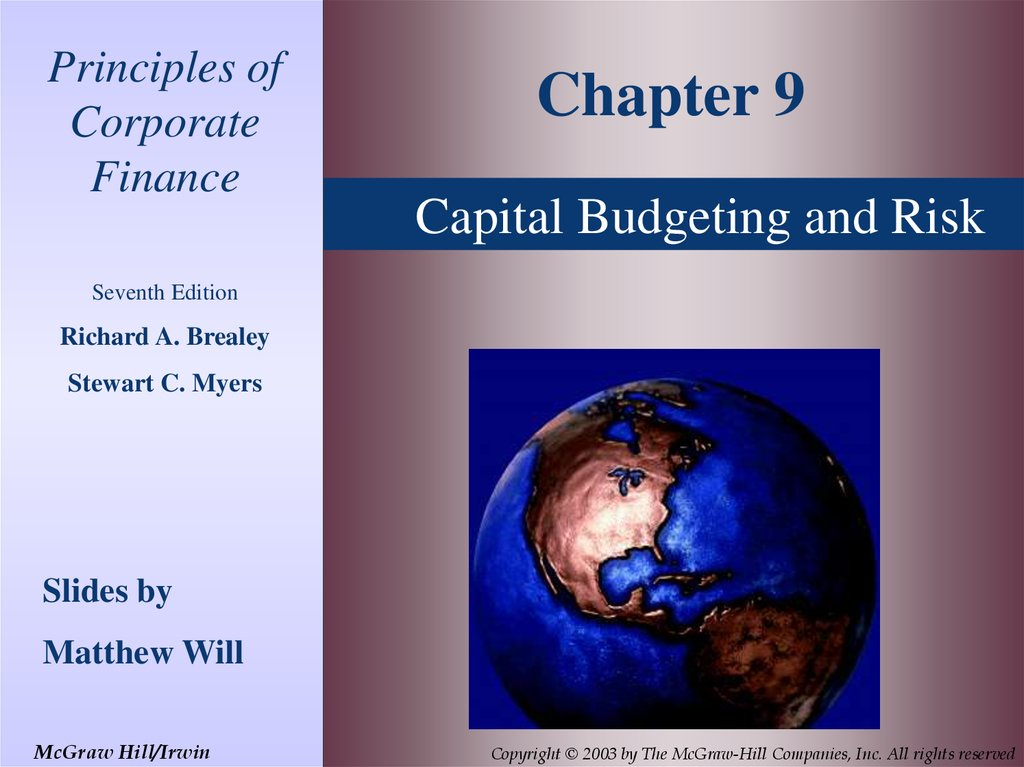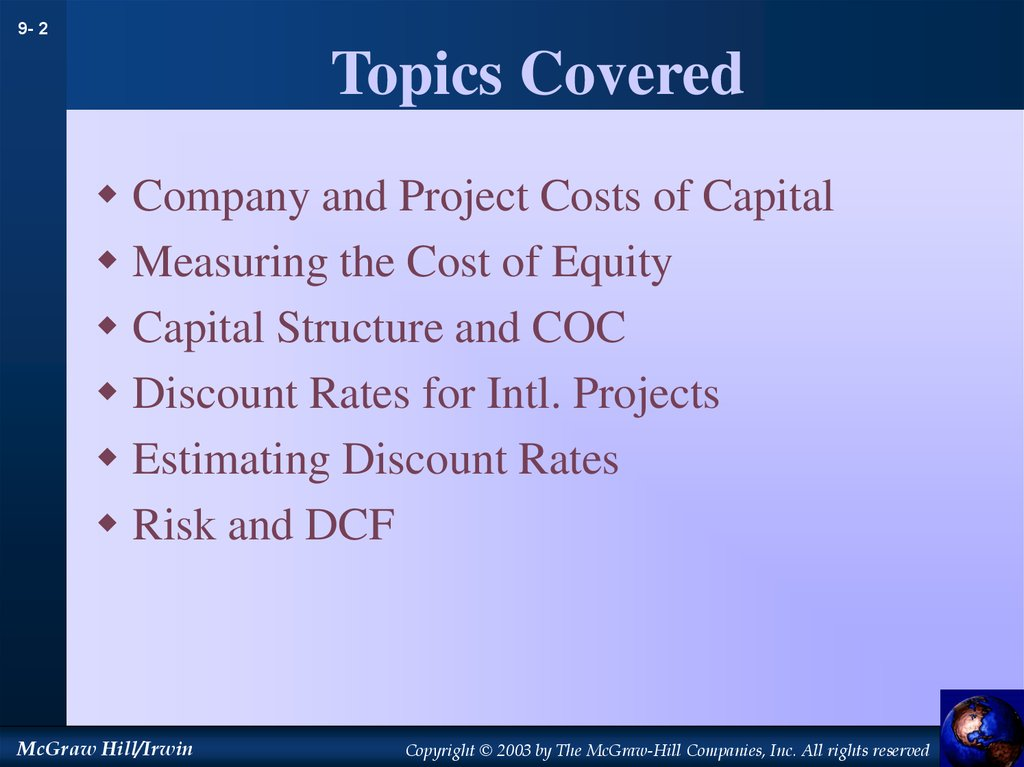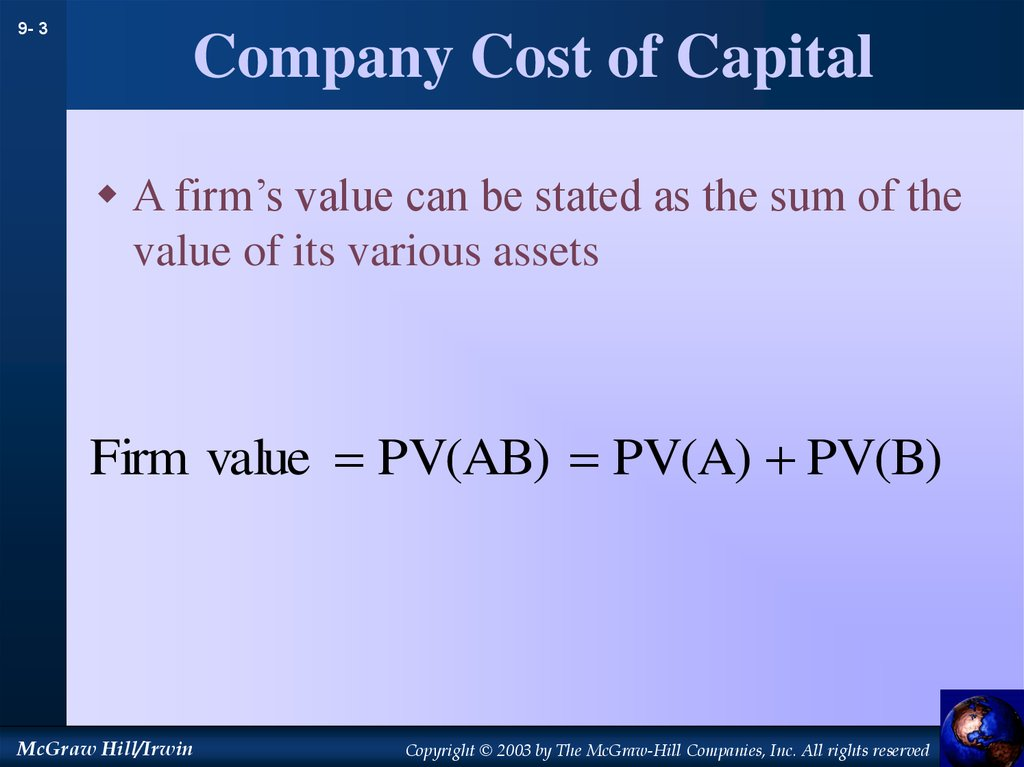# Capital Budgeting and Risk

## 1.

Principles of
Corporate
Finance
Chapter 9
Capital Budgeting and Risk
Seventh Edition
Richard A. Brealey
Stewart C. Myers
Slides by
Matthew Will
McGraw Hill/Irwin

## 2. Topics Covered

9- 2
Topics Covered
Company and Project Costs of Capital
Measuring the Cost of Equity
Capital Structure and COC
Discount Rates for Intl. Projects
Estimating Discount Rates
Risk and DCF
McGraw Hill/Irwin

## 3. Company Cost of Capital

9- 3
Company Cost of Capital
A firm’s value can be stated as the sum of the
value of its various assets
Firm value PV(AB) PV(A) PV(B)
McGraw Hill/Irwin

## 4. Company Cost of Capital

9- 4
Company Cost of Capital
Category
Discount Rate
Speculativ e ventures
30%
New products
20%
15% (Company COC)
Cost improvemen t, known tech nology
10%
McGraw Hill/Irwin

## 5. Company Cost of Capital

9- 5
Company Cost of Capital
A company’s cost of capital can be compared
to the CAPM required return
SML
Required
return
13
Company Cost
of Capital
5.5
0
1.26
McGraw Hill/Irwin
Project Beta

## 6. Measuring Betas

9- 6
Measuring Betas
The SML shows the relationship between
return and risk
CAPM uses Beta as a proxy for risk
Other methods can be employed to determine
the slope of the SML and thus Beta
Regression analysis can be used to find Beta
McGraw Hill/Irwin

## 7. Measuring Betas

9- 7
Measuring Betas
Dell Computer
Dell return (%)
Price data – Aug 88- Jan 95
R2 = .11
B = 1.62
Slope determined from plotting the
line of best fit.
McGraw Hill/Irwin
Market return (%)

## 8. Measuring Betas

9- 8
Measuring Betas
Dell Computer
Dell return (%)
Price data – Feb 95 – Jul 01
R2 = .27
B = 2.02
Slope determined from plotting the
line of best fit.
McGraw Hill/Irwin
Market return (%)

## 9. Measuring Betas

9- 9
Measuring Betas
General Motors
GM return (%)
Price data – Aug 88- Jan 95
R2 = .13
B = 0.80
Slope determined from plotting the
line of best fit.
McGraw Hill/Irwin
Market return (%)

## 10. Measuring Betas

9- 10
Measuring Betas
General Motors
GM return (%)
Price data – Feb 95 – Jul 01
R2 = .25
B = 1.00
Slope determined from plotting the
line of best fit.
McGraw Hill/Irwin
Market return (%)

## 11. Measuring Betas

9- 11
Measuring Betas
Exxon Mobil
Exxon Mobil return (%)
Price data – Aug 88- Jan 95
R2 = .28
B = 0.52
Slope determined from plotting the
line of best fit.
McGraw Hill/Irwin
Market return (%)

## 12. Measuring Betas

9- 12
Measuring Betas
Exxon Mobil
Exxon Mobil return (%)
Price data – Feb 95 – Jul 01
R2 = .16
B = 0.42
Slope determined from plotting the
line of best fit.
McGraw Hill/Irwin
Market return (%)

## 13. Beta Stability

9- 13
Beta Stability
RISK
CLASS
% IN SAME
CLASS 5
YEARS LATER
% WITHIN ONE
CLASS 5
YEARS LATER
10 (High betas)
35
69
9
18
54
8
16
45
7
13
41
6
14
39
5
14
42
4
13
40
3
16
45
2
21
61
1 (Low betas)
40
62
Source: Sharpe and Cooper (1972)
McGraw Hill/Irwin

## 14. Company Cost of Capital simple approach

9- 14
Company Cost of Capital
simple approach
Company Cost of Capital (COC) is based on
the average beta of the assets
The average Beta of the assets is based on the
% of funds in each asset
McGraw Hill/Irwin

## 15. Company Cost of Capital simple approach

9- 15
Company Cost of Capital
simple approach
Company Cost of Capital (COC) is based on the average beta of
the assets
The average Beta of the assets is based on the % of funds in
each asset
Example
1/3 New Ventures B=2.0
1/3 Plant efficiency B=0.6
AVG B of assets = 1.3
McGraw Hill/Irwin

## 16. Capital Structure

9- 16
Capital Structure
Capital Structure - the mix of debt & equity within a company
Expand CAPM to include CS
R = rf + B ( rm - rf )
becomes
Requity = rf + B ( rm - rf )
McGraw Hill/Irwin

## 17. Capital Structure & COC

9- 17
Capital Structure & COC
COC = rportfolio = rassets
rassets = WACC = rdebt (D) + requity (E)
(V)
(V)
Bassets = Bdebt (D) + Bequity (E)
(V)
(V)
requity = rf + Bequity ( rm - rf )
McGraw Hill/Irwin
IMPORTANT
E, D, and V are
all market values

## 18. Capital Structure & COC

9- 18
Capital Structure & COC
Expected Returns and Betas prior to refinancing
Expected
return (%)
20
Requity=15
Rassets=12.2
Rrdebt=8
0
0
McGraw Hill/Irwin
0.2
0.8
Bdebt
Bassets
1.2
Bequity

## 19. Union Pacific Corp.

9- 19
Union Pacific Corp.
Requity = Return on Stock
= 15%
Rdebt = YTM on bonds
= 7.5 %
McGraw Hill/Irwin

## 20. Union Pacific Corp.

9- 20
Union Pacific Corp.
Beta
McGraw Hill/Irwin
Standard. Error
Burlington Northern
.64
.20
CSX Transporta tion
.46
.24
Norfolk Southern
.52
.26
Union Pacific
.40
.21
Industry Portfolio
.50
.17

## 21. Union Pacific Corp.

9- 21
Union Pacific Corp.
Example
Assets
100
Total Assets
Rassets
Rassets
McGraw Hill/Irwin
Debt value
30
Equity val ue
70
Firm value
100
debt
equity
rdebt
requity
debt equity
debt equity
30
70
7.5%
15% 12.75%
30 70
30 70

## 22. International Risk

9- 22
International Risk
Ratio
Correlatio n
Beta
Egypt
3.11
coefficien t
.18
Poland
1.93
.42
0.81
Thailand
2.91
.48
1.39
Venezuela
2.58
.30
0.77
0.55
Source: The Brattle Group, Inc.
Ratio - Ratio of standard deviations, country index vs. S&P composite
index
McGraw Hill/Irwin

## 23. Asset Betas

9- 23
B revenue
Asset Betas
PV(fixed cost)
Bfixed cost
PV(revenue )
PV(variabl e cost)
PV(asset)
B variable cost
Basset
PV(revenue )
PV(revenue )
McGraw Hill/Irwin

## 24. Asset Betas

9- 24
Asset Betas
Basset B revenue
PV(revenue ) - PV(variabl e cost)
PV(asset)
PV(fixed cost)
B revenue 1
PV(asset)
McGraw Hill/Irwin

## 25. Risk,DCF and CEQ

9- 25
Risk,DCF and CEQ
Ct
CEQt
PV
t
t
(1 r )
(1 rf )
McGraw Hill/Irwin

## 26. Risk,DCF and CEQ

9- 26
Risk,DCF and CEQ
Example
Project A is expected to produce CF = \$100 mil for
each of three years. Given a risk free rate of 6%, a
market premium of 8%, and beta of .75, what is the
PV of the project?
McGraw Hill/Irwin

## 27. Risk,DCF and CEQ

9- 27
Risk,DCF and CEQ
Example
Project A is expected to produce CF = \$100 mil for each of three years.
Given a risk free rate of 6%, a market premium of 8%, and beta of .75,
what is the PV of the project?
r rf B( rm rf )
6 .75(8)
12%
McGraw Hill/Irwin

## 28. Risk,DCF and CEQ

9- 28
Risk,DCF and CEQ
Example
Project A is expected to produce CF = \$100 mil for each of three years.
Given a risk free rate of 6%, a market premium of 8%, and beta of .75,
what is the PV of the project?
Project A
r rf B( rm rf )
6 .75(8)
12%
McGraw Hill/Irwin
Year
Cash Flow
PV @ 12%
1
100
89.3
2
100
79.7
3
100
71.2
Total PV
240.2

## 29. Risk,DCF and CEQ

9- 29
Risk,DCF and CEQ
Example
Project A is expected to produce CF = \$100 mil for each of three years.
Given a risk free rate of 6%, a market premium of 8%, and beta of .75,
what is the PV of the project?
Project A
Year
Cash Flow
PV @ 12%
1
100
89.3
2
100
79.7
3
100
71.2
Total PV
240.2
r rf B( rm rf )
Now assume that the cash
flows change, but are
RISK FREE. What is the
new PV?
6 .75(8)
12%
McGraw Hill/Irwin

## 30. Risk,DCF and CEQ

9- 30
Risk,DCF and CEQ
Example
Project A is expected to produce CF = \$100 mil for each of three years.
Given a risk free rate of 6%, a market premium of 8%, and beta of .75,
what is the PV of the project?.. Now assume that the cash flows change,
but are RISK FREE. What is the new PV?
Project B
Project A
Year
Cash Flow
PV @ 6%
1
94.6
89.3
Year
Cash Flow
PV @ 12%
1
100
89.3
2
100
79.7
2
89.6
79.7
3
100
71.2
3
84.8
71.2
Total PV
240.2
Total PV
240.2
McGraw Hill/Irwin

## 31. Risk,DCF and CEQ

9- 31
Risk,DCF and CEQ
Example
Project A is expected to produce CF = \$100 mil for each of three years.
Given a risk free rate of 6%, a market premium of 8%, and beta of .75,
what is the PV of the project?.. Now assume that the cash flows change,
but are RISK FREE. What is the new PV?
Project B
Project A
Year
Cash Flow
PV @ 12%
Year
Cash Flow
PV @ 6%
1
100
89.3
1
94.6
89.3
2
100
79.7
2
89.6
79.7
3
100
71.2
3
84.8
71.2
Total PV
240.2
Total PV
240.2
Since the 94.6 is risk free, we call it a Certainty Equivalent
of the 100.
McGraw Hill/Irwin

## 32. Risk,DCF and CEQ

9- 32
Risk,DCF and CEQ
Example
Project A is expected to produce CF = \$100 mil for each of three years.
Given a risk free rate of 6%, a market premium of 8%, and beta of .75,
what is the PV of the project? DEDUCTION FOR RISK
Year Cash Flow
McGraw Hill/Irwin
CEQ
Deduction
1
100
94.6
for risk
5.4
2
100
89.6
10.4
3
100
84.8
15.2

## 33. Risk,DCF and CEQ

9- 33
Risk,DCF and CEQ
Example
Project A is expected to produce CF = \$100 mil for each of three years.
Given a risk free rate of 6%, a market premium of 8%, and beta of .75,
what is the PV of the project?.. Now assume that the cash flows change,
but are RISK FREE. What is the new PV?
The difference between the 100 and the certainty equivalent
(94.6) is 5.4%…this % can be considered the annual
premium on a risky cash flow
Risky cash flow
certainty equivalent cash flow
1.054
McGraw Hill/Irwin

## 34. Risk,DCF and CEQ

9- 34
Risk,DCF and CEQ
Example
Project A is expected to produce CF = \$100 mil for each of three years.
Given a risk free rate of 6%, a market premium of 8%, and beta of .75,
what is the PV of the project?.. Now assume that the cash flows change,
but are RISK FREE. What is the new PV?
100
Year 1
94.6
1.054
Year 2
100
89.6
2
1.054
100
Year 3
84.8
3
1.054
McGraw Hill/Irwin